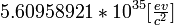# Mass

In physics, mass is an extensive physical property of a system and is most frequently measured in the SI unit of kilograms. Mass is the the "charge" of the gravitational force, and the resistance an object has to force. The first form of mass is called gravitational mass; the second inertial mass. While most physical theories do not require gravitational mass and inertial mass to be equal, no object or condition has ever been found where the two are not equal, and most discussions simplify by using only the term mass.

Inertial mass is the measure of an object's propensity (or lack thereof) to accelerate when a force is applied to it as given by Newton's Second Law: F = ma, and thus m = F/a, where F is force, a is acceleration, and m is mass.

As the "charge" of the gravitational force, any object possessing mass exerts a force on any other object possessing mass; in Newtonian mechanics, that force is given as:$F = G\, \frac{M_{\mathrm{1}}M_{\mathrm{2}}}{R^2_{\mathrm{}}}$, where F is force, G is the gravitational constant, and R is the distance between the two objects.

##  Mass versus weight

Mass is frequently confused with weight which is actually a measure of the gravitational force applied to a mass. This is why you can be "lighter weight" on the moon without having a lower mass. This problem occurs frequently when using U.S. customary units because the U.S. system uses pounds which is a unit of force as a basic unit, while the SI uses kilograms, a unit of mass, as a basic unit.

##  Units of mass

The basic unit of mass is SI is the kilogram (abbreviated kg), the standard of mass is the International Prototype Kilogram .

1 kg (SI) = 0.0685 [slug] (English)

1 kg (SI) =$5.60958921*10^{35} \lbrack \tfrac{ev}{c^2} \rbrack$ where "ev" is the unit electron-volt and c is the speed of light. The "c²" is often dropped when using this unit.

1 kg (SI) = 1.67386428 * 10 − 25 emu Earth mass units

1 kg (SI) = 5.02785431 * 10 − 31 solar masses

##  Gravitational versus inertial mass

The fact that the same quantity serves as both the "charge" for the gravitational force and the inertial term of Newton's Second Law is neither necessary nor predicted by other laws of physics. This observed fact has led to some open problems in gravity. Albert Einstein assumed it to be true in his formulation of General Relativity.

##  Relativistic Mass

In relativistic physics, mass and energy are interchangeable, as described by Einstein's famous equation E=mc². Consequences of this are that energy exerts gravitational force, and that electromagnetic radiation is affected by gravity.Some content on this page may previously have appeared on Citizendium.